#### Resolution issues

One of the main complications in using state discretization is that there are three spaces over which sampling occurs: time, the action space, and the state space. Assume the discrete-time model is used. If obtaining optimal solutions is important, then very small cells should be used (e.g., 50 to 100 per axis). This limits its application to state spaces of a few dimensions. The time interval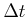should also be made small, but if it is too small relative to the cell size, then it may be impossible to leave a cell. If only feasibility is the only requirement, then larger cells may be used, andmust be appropriately increased. A course quantization of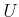may cause solutions to be missed, particularly ifis large. Asdecreases, the number of samples in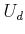becomes less important.

To obtain resolution completeness, the sampling should be improved each time the search fails. Each time that the search is started, the sampling dispersion for at least one of the three spaces should be decreased. The possibilities are 1) the time intervalmay be reduced, 2) more actions may be added to, or 3) more points may be added toto reduce the cell size. If the dispersion approaches zero for all three spaces, and if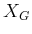contains an open subset of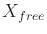, then resolution completeness is obtained. Ifis only a point, then solutions that come within some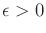must be tolerated.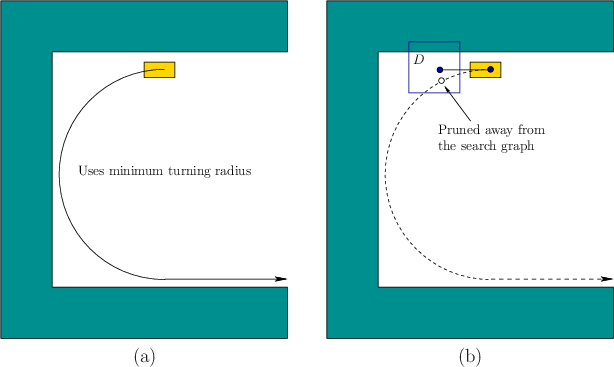Recall that resolution completeness assumes that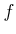has bounded derivatives or at least satisfies a Lipschitz condition (14.11). The actual rate of convergence is mainly affected by three factors: 1) the rate at whichvaries with respect to changes inand(characterized by Lipschitz constants), 2) the required traversal of narrow regions in, and 3) the controllability of the system. The last condition will be studied further for nonholonomic systems in Section 15.4. For a concrete example, consider making a U-turn with a Dubins car that has a very large turning radius, as shown in Figure 14.18. A precise turn may be required to turn around, and this may depend on an action that was chosen many stages earlier. The Dubins car model does not allow zig-zagging (e.g., parallel parking) maneuvers to make local corrections to the configuration.

Steven M LaValle 2020-08-14